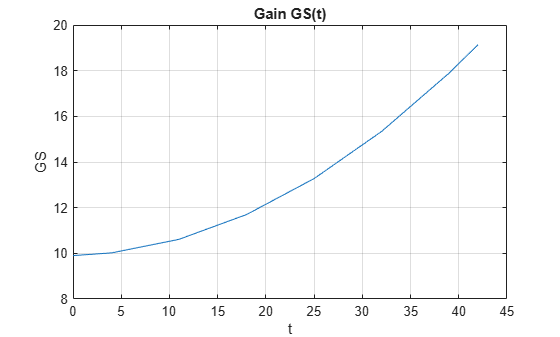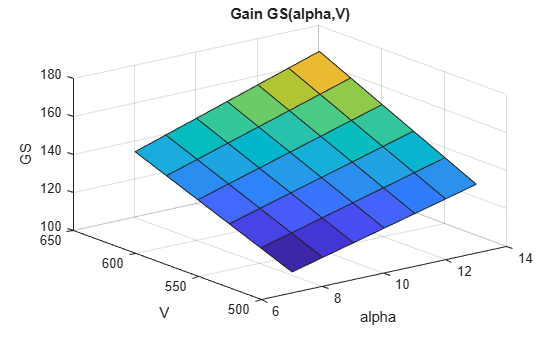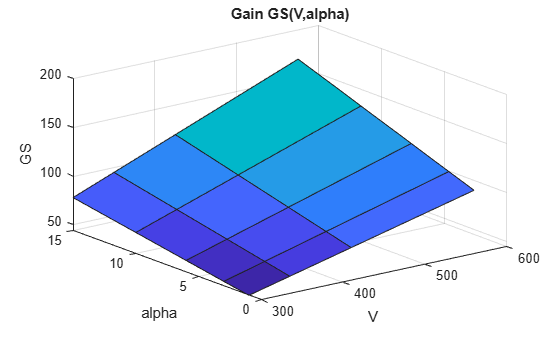# viewSurf

Visualize gain surface as a function of scheduling variables

## Syntax

``viewSurf(GS)``
``view(GS,xvar,xdata)``
``view(GS,xvar,xdata,yvar,ydata)``

## Description

example

````viewSurf(GS)` plots the values of a 1-D or 2-D gain surface as a function of the scheduling variables. `GS` is a tunable gain surface that you create with `tunableSurface`. The plot uses the independent variable values specified in `GS.SamplingGrid`. For 2-D gain surfaces, the design points in `GS.SamplingGrid` must lie on a rectangular grid.```

example

````view(GS,xvar,xdata)` plots the gain surface `GS` at the scheduling-variable values listed in `xdata`. The variable name `xvar` must match a scheduling variable name in `GS.SamplingGrid`. However, the values in `xdata` need not match design points in `GS.SamplingGrid`.For a 2-D gain surface, the plot shows a parametric family of curves with one curve per value of the other scheduling variable. In the 2-D case, the design points in `GS.SamplingGrid` must lie on a rectangular grid.```

example

````view(GS,xvar,xdata,yvar,ydata)` creates a surface plot of a 2-D gain surface evaluated over a grid of scheduling variable values given by `ndgrid(xdata,ydata)`. In this case, the design points of `GS` do not need to lie on a rectangular grid, and `xdata` and `ydata` do not need to match the design points.```

## Examples

collapse all

Display a tunable gain surface that depends on two independent variables.

Model a scalar gain K with a bilinear dependence on two scheduling variables, $\alpha$ and V, as follows:

`$K\left(\alpha ,V\right)={K}_{0}+{K}_{1}x+{K}_{2}y+{K}_{3}xy.$`

Here, x and y are the normalized scheduling variables. Suppose that $\alpha$ is an angle of incidence that ranges from 0 degrees to 15 degrees, and V is a speed that ranges from 300 m/s to 600 m/s. Then, x and y are given by:

`$x=\frac{\alpha -7.5}{7.5},\phantom{\rule{1em}{0ex}}y=\frac{V-450}{150}.$`

The coefficients ${K}_{0},...,{K}_{3}$ are the tunable parameters of this variable gain. Use `tunableSurface` to model this variable gain.

```[alpha,V] = ndgrid(0:1.5:15,300:30:600); domain = struct('alpha',alpha,'V',V); shapefcn = @(x,y) [x,y,x*y]; K = tunableSurface('K',1,domain,shapefcn);```

Typically, you would tune the coefficients as part of a control system. You would then use `setBlockValue` or `setData` to write the tuned coefficients back to `K`, and view the tuned gain surface. For this example, instead of tuning, manually set the coefficients to non-zero values and view the resulting gain.

```Ktuned = setData(K,[100,28,40,10]); viewSurf(Ktuned)````viewSurf` displays the gain surface as a function of the scheduling variables, for the ranges of values specified by `domain` and stored in `Ktuned.SamplingGrid`.

View a 1-D gain surface evaluated at different design points from the points specified in the gain surface.

When you create a gain surface using `tunableSurface`, you specify design points at which the gain coefficients are tuned. These points are the typically the scheduling-variable values at which you have sampled or linearized the plant. However, you might want to implement the gain surface as a lookup table with breakpoints that are different from the specified design points. In this example, you create a gain surface with a set of design points and then view the surface using a different set of scheduling variable values.

Create a scalar gain that varies as a quadratic function of one scheduling variable, t. Suppose that you have linearized your plant every five seconds from t = 0 to t = 40.

```t = 0:5:40; domain = struct('t',t); shapefcn = @(x) [x,x^2]; GS = tunableSurface('GS',1,domain,shapefcn);```

Typically, you would tune the coefficients as part of a control system. For this example, instead of tuning, manually set the coefficients to non-zero values.

`GS = setData(GS,[12.1,4.2,2]);`

Plot the gain surface evaluated at a different set of time values.

```tvals = [0,4,11,18,25,32,39,42]; viewSurf(GS,'t',tvals)```The plot shows that the gain curve bends at the points specified in `tvals`, rather than the design points specified in `domain`. Also, `tvals` includes values outside of the scheduling-variable range of `domain`. If you attempt to extrapolate too far out of the range of values used for tuning, the software issues a warning.

Plot gain surface values as a function of one independent variable, for a gain surface that depends on two independent variables.

Create a gain surface that is a bilinear function of two independent variables, $\alpha$ and V.

```[alpha,V] = ndgrid(0:1.5:15,300:30:600); domain = struct('alpha',alpha,'V',V); shapefcn = @(x,y) [x,y,x*y]; GS = tunableSurface('GS',1,domain,shapefcn);```

Typically, you would tune the coefficients as part of a control system. For this example, instead of tuning, manually set the coefficients to non-zero values.

`GS = setData(GS,[100,28,40,10]);`

Plot the gain at selected values of V.

```Vplot = [300:50:600]; viewSurf(GS,'V',Vplot);````viewSurf` evaluates the gain surface at the specified values of V, and plots the dependence on V for all values of $\alpha$ in `domain`. Clicking any of the lines in the plot displays the corresponding $\alpha$ value. This plot is useful to visualize the full range of gain variation due to one independent variable.

View a 2-D gain surface evaluated at different scheduling-variable values from the design points specified in the gain surface.

When you create a gain surface using `tunableSurface`, you specify design points at which the gain coefficients are tuned. These points are the typically the scheduling-variable values at which you have sampled or linearized the plant. However, you might want to implement the gain surface as a lookup table with breakpoints that are different from the specified design points. In this example, you create a gain surface with a set of design points and then view the surface using a different set of scheduling-variable values.

Create a gain surface that is a bilinear function of two independent variables, $\alpha$ and V.

```[alpha,V] = ndgrid(0:1.5:15,300:30:600); domain = struct('alpha',alpha,'V',V); shapefcn = @(x,y) [x,y,x*y]; GS = tunableSurface('GS',1,domain,shapefcn);```

Typically, you would tune the coefficients as part of a control system. For this example, instead of tuning, manually set the coefficients to non-zero values.

`GS = setData(GS,[100,28,40,10]);`

Plot the gain at selected values of $\alpha$ and V.

```alpha_vec = [7:1:13]; V_vec = [500:25:625]; viewSurf(GS,'alpha',alpha_vec,'V',V_vec);```The breakpoints at which you evaluate the gain surface need not fall within the range specified by `domain`. However, if you attempt to evaluate the gain too far outside the range used for tuning, the software issues a warning.

The breakpoints also need not be regularly spaced. In addition, you can specify the scheduling variables in any order to get a different perspective on the shape of the surface. The variable that you specify first is used as the X-axis in the plot.

```alpha_vec2 = [1,3,6,10,15]; V_vec2 = [300,350,425,575]; viewSurf(GS,'V',V_vec2,'alpha',alpha_vec2);```## Input Arguments

collapse all

Gain surface to plot, specified as a `tunableSurface` object. `GS` can depend on one or two scheduling variables, and must be scalar-valued.

X-axis variable in the plot, specified as a character vector. The variable name `xvar` must match a scheduling variable name in `GS.SamplingGrid`.

X-axis-variable values at which to evaluate and plot the gain surface, specified as a numeric vector.

Y-axis variable in the plot, specified as a character vector. The variable name `yvar` must match a scheduling variable name in `GS.SamplingGrid`.

Y-axis-variable values at which to evaluate and plot the gain surface, specified as a numeric vector.